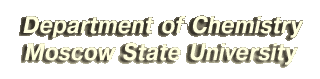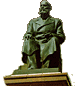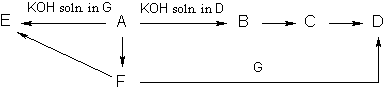# Example Problems for Entrance Examinations

## Preliminary round

Problem 1.

Give an example of chemical reaction between a salt and an acid leading to the formation of another salt and a base.
(1 point) [Solution]

Problem 2.

Write the reactions involved in the following sequence of oxidation number transformations:
(2 points) [Solution]

P0P-3P-3P+5P+5

Problem 3.

Write the reactions of chromium (III) bromide with hydrogen peroxide in acidic and basic media.
(2 points) [Solution]

Problem 4.

Write the reactions involved in the following sequence:

C10H12C3H6O2C3H5OClC10H12O2.

In the reactions, specify the structures of all substances.
(3 points) [Solution]

Problem 5.

Write the reactions involved in the following sequence of transformations:Determine the unknown substances.
(4 points) [Solution]

Problem 6.

Three substances A, B, and C were mixed in the amount of three moles each. When the equilibrium
A + B2C
was reached, five moles of substance C were found in the system. Calculate the equilibrium constant. Determine the equilibrium composition (in mol.%) of mixture, obtained by adding three moles of A and two moles of B to one mole of C at the same temperature.
(4 points) [Solution]

Problem 7.

Combustion of 10.7 g of unknown substance gave 30.8 g of CO2, 8.1 g of Í2O, and 1.4 g of N2. The complete hydrogenation of the same sample of this substance required 9.0 l of hydrogen at temperature 227 oC and pressure 138.5 kPa. The gas obtained by complete evaporation of 3.21 g of the substance occupied the volume of 1.25 l at temperature 227 oC and pressure 99.7 kPa. Draw the four possible structures of the unknown substance.
(4 points) [Solution]

• Example problems in chemistry for the entrance exams (main round)
• Special Program developed for chemistry entrance examinations in Moscow State University.

Chemistry Department of Moscow State UniversityThe site is supported by Russian Foundation for Basic Research
The using of published on this page materials is not allowed without special permission
Copyright (C) Chemisty Department of Moscow State University
Web-Editor: B.I.Pokrovskii
Web-design: Copyright (C) I. Minyaylova and V. Minaylov
webmaster@www.chem.msu.su